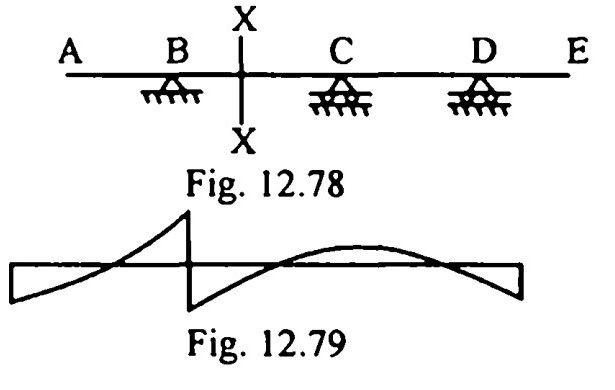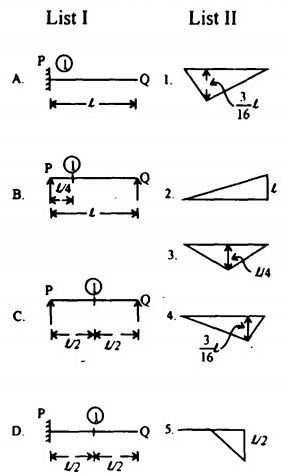top of pageSearch

# Structural Analysis (161-170)

Updated: Apr 30, 2020

161.For beam shown in Fig. 12.78, an influences line diagram is shown in Fig. This refers to shear force at section XX

162.163. The beam shown in Fig. 12.81 carries loads of 20 kN and 40 kN at points 'X'

and 'Y' respectively and produces a deflection of 6 mm at point ZTo produce deflections of 8mm and 5 mm at 'X' and 'Y' respectively, the load

required at Z would be 60 kN

164.A portal frame is shown in Fig. For EI constant, the deflected shape of the

frame will be as ina) Both A and R are true and R is the correct explanation of A.

b) Both A and R are true but R is not the correct explanation of A.

c) A is true but R is false

d) A is false but R is true

165

Assertion A : When the system of loads shown in Fig. crosses a simple span

of any length, the absolute maximum bending moment occurs at the mid-span

when the middle load stays at mid-spanReason R : The resultant load of the system coincides with the middle load

a

166. Assertion A : The concept of strain energy can be used to analyse a statically

indetermine structure.

Reason R : There is a direct relationship between strain energy of a structure and

the slopes and deflection caused in it.

a

167. Assertion A : In the analysis of rigid frames, the usual practice is to consider

the strain energy due to flexure only.

Reason R : The strain energies due to axial and shear forces are usually quite

small compared to that of flexure.

a

168. Assertion A : Whether it is maximum BM at a section or absolute maximum BM, the moving UDL should cover the entire span of a simple beam if span is less than load length.

Reason R : Whether it is maximum BM at a section or absolute maximum BM, the moving UDL should be divided by the section in the same ratio in which the

section divides the span, if the span is greater than load length.

b

169.Assertion A : Influence Line Diagram (ILD) for S.F. at the fixed end of a

cantilever and SFD due to unit load at the free end are same.

Reason R : ILD for BM at the fixed endof a cantilever and BMD due to unit load

at the free end are same. c

170.Assertion A : When a single load crosses a simple span of any length, the shear

force under the load to the right for all spans is constant, so long as the load

maintains the1 same all ratio (see figure

)Reason R : When the load maintains the same all ratio in all spans, the reaction at

the right hand support remains the same a